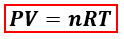# Problem: What pressure will 14.0 g of CO exert in a 3.5 L container at 75 degrees Celsius?

🤓 Based on our data, we think this question is relevant for Professor Dixon's class at UCF.

###### FREE Expert Solution

We are asked to find the pressure of CO at the given conditions. Since mass, volume and temperature are given, we will use the ideal gas equation to calculate for pressure.P = pressure, atm
V = volume, L
n = moles, mol
R = gas constant = 0.08206 (L·atm)/(mol·K)
T = temperature, K

In the ideal gas equation, moles are needed but the mass of CO is given instead. We’ll calculate the moles of CO using the mass and its molar mass.###### Problem Details

What pressure will 14.0 g of CO exert in a 3.5 L container at 75 degrees Celsius?

What scientific concept do you need to know in order to solve this problem?

Our tutors have indicated that to solve this problem you will need to apply the The Ideal Gas Law concept. You can view video lessons to learn The Ideal Gas Law. Or if you need more The Ideal Gas Law practice, you can also practice The Ideal Gas Law practice problems.

What is the difficulty of this problem?

Our tutors rated the difficulty ofWhat pressure will 14.0 g of CO exert in a 3.5 L container a...as medium difficulty.

How long does this problem take to solve?

Our expert Chemistry tutor, Dasha took 4 minutes undefined to solve this problem. You can follow their steps in the video explanation above.

What professor is this problem relevant for?

Based on our data, we think this problem is relevant for Professor Dixon's class at UCF.